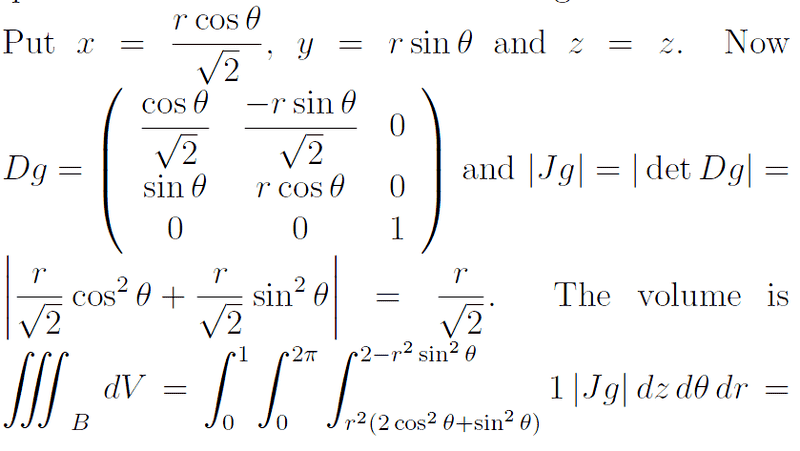# Triple integral & cylindrical coordinates

## Homework Statement

When you are doing a triple integral and convert it to cylindrical co ordinates, how do you find the new ranges of integration?

I understand the new range of z, if z is between f(x,y) and g(x,y), you just sub in
x = r cos θ and y = r sin θ to find the new functions. But how do I find the range for r and θ? I'm lost there.

Here is a question i was working on and the solution is a bit confusing.

Find the volume of the solid bounded by the paraboloid z = 4x^2 +y^2 and the cylinder
y^2 + z = 2.

So the solution, they replace x = r cos θ / root 2 and y = r sin θ. I'm confused why not just use x = r cos θ? and the range for r is from 0 to 1 and for θ its between 0 and 2pi.

## The Attempt at a Solution

SammyS
Staff Emeritus
Homework Helper
Gold Member
Can you show, or explain completely, the triple integral which is given as the solution?

Here is the portion of the solution where they derive the integral.vela
Staff Emeritus
Homework Helper
Find the equation x and y satisfy where the two surfaces intersect. From that, you might be able to see why x was rescaled the way it was in the solution.

SammyS
Staff Emeritus
Homework Helper
Gold Member

## Homework Statement

When you are doing a triple integral and convert it to cylindrical co ordinates, how do you find the new ranges of integration?

I understand the new range of z, if z is between f(x,y) and g(x,y), you just sub in
x = r cos θ and y = r sin θ to find the new functions. But how do I find the range for r and θ? I'm lost there.

Here is a question i was working on and the solution is a bit confusing.

Find the volume of the solid bounded by the paraboloid z = 4x^2 +y^2 and the cylinder
y^2 + z = 2.

So the solution, they replace x = r cos θ / root 2 and y = r sin θ. I'm confused why not just use x = r cos θ? and the range for r is from 0 to 1 and for θ its between 0 and 2pi.
...

Why does their solution "replace x = r cos θ / root 2 and y = r sin θ" ?

It's as if they first rescale x: Letting x' = (√2)x. Note: x' is not a derivative.

Then x' = r cos(θ) and y = r sin(θ) .

The reason they did this is explained in vela's post.

Thanks.

Find the equation x and y satisfy where the two surfaces intersect. From that, you might be able to see why x was rescaled the way it was in the solution.

So its 4x^2 + 2y^2 < 2

But I still don't understand? How would you know to rescale it by multiplying it by 1/sqrt 2?
What does this rescaling do? I'm still a bit lost.

And how do I find the ranges for r and theta?
Does θ vary based on the quadrant or what?

SammyS
Staff Emeritus
Homework Helper
Gold Member
Thanks.

So its 4x^2 + 2y^2 < 2

But I still don't understand? How would you know to rescale it by multiplying it by 1/sqrt 2?
What does this rescaling do? I'm still a bit lost.

And how do I find the ranges for r and theta?
Does θ vary based on the quadrant or what?
Can you identify what geometric figure the equation 4x2 + 2y2 = 2 describes?

Suppose x' = (√2)x, i.e. x = x'/√2 . Substitute that into this equation. What figure is now described by the result?

Can you identify what geometric figure the equation 4x2 + 2y2 = 2 describes?

Suppose x' = (√2)x, i.e. x = x'/√2 . Substitute that into this equation. What figure is now described by the result?

It's a circle with r = 1, which I guess is how you get the range of r; with that substitution rather than an ellipse. I wasn't aware you are allowed to just make arbitrary substitutions like that to change the shape.

SammyS
Staff Emeritus